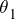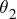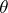# Day 154 physics

Refraction is the bending of a wave when it encounters a boundary between two different media

• All types of waves exhibit refraction; here we will be concerned with the behavior of light.
• In the 1600s, Newton originally thought that light traveled slower in a medium with a smaller density.
• Snell proved Newton wrong since light travels the fastest in a vacuum and the slowest in a medium with a high optical density.

## Refraction Diagrams

• This law is based on the angle of incidence (previously discussed with reflection), the angle of refraction and the normal.
• The angle of refraction is the angle between the refracted ray and the normal. Remember, the normal is the perpendicular to the boundary.

## What Determines the Angle of Refraction?

• When a light moves from a less to more dense material, it slows down and bends toward the normal
• Therefore, the angle of refraction is smaller than the angle of incidence The converse is also true: when moving from a more to less dense material, the light bends away from the normal and the angle of refraction becomes larger than the angle of incidence.

## Index of Refraction

Every material has its own index of refraction based on how fast light travels through it. The greater the index of refraction, n, the greater its optical density

• No material can have a value of n less than 1.00
• n for a vacuum is equal to 1.00
• Values can be obtained by looking in a reference book for an index of refraction table
• n = c/v=speed in vacuum/speed in substance
• You should be able to see why n can never be less than one, since light always slows down when it encounters a material Also, n has no units, since they would cancel in the above formula

## Snell’s Law

•sin=sin•= index of refraction of the incident medium (medium 1)= index of refraction of the refraction medium (medium 2)
•= angle of incidence
•= angle of refraction

## Important Points

• Remember that when the speed of wave changes, either the frequency or the wavelength must also change.
• If the source has NOT changed, as in most cases, the frequency CANNOT change. Therefore, the wavelength is the other variable that changes along with the speed

## Light Through a Prism

• Everyone has seen (in person or by picture), light passing through a prism ¬Since white light is made up of different wavelengths, the amount of bending varies along with this wavelength. Longer wavelengths (red) tend to bend more than shorter wavelengths (violet)
• Therefore, the characteristic “rainbow” effect

## Critical Angle

• Each material has a value, the critical angle,c, which can be the incident angle.
• If the incident angle is equal to the critical angle, light refracts along the boundary between the two materials
• In this case,2= 90°.
• When the incident angle is above this angle, the light ray reflects rather than refracts and the first law of reflection applies
• This is called internal reflection

Internal reflection can only occur when light is passing from a greater to a smaller density material. It is physically impossible for it to occur with the media reversed. If the light can never escape, like in a fiber optic tube, it is called total internal reflection

## Diagram of Scenario(source)